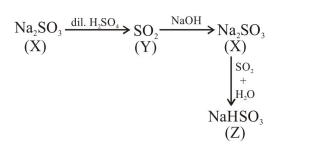# Reaction of an inorganic sulphite X with dilute

Question:

Reaction of an inorganic sulphite $\mathrm{X}$ with dilute $\mathrm{H}_{2} \mathrm{SO}_{4}$ generates compound $\mathrm{Y}$. Reaction of $\mathrm{Y}$ with $\mathrm{NaOH}$ gives $X$. Further, the reaction of $X$ with $\mathrm{Y}$ and water affords compound $\mathrm{Z}$. $\mathrm{Y}$ and $Z$, respectively, are:

1. $\mathrm{S}$ and $\mathrm{Na}_{2} \mathrm{SO}_{3}$

2. $\mathrm{SO}_{2}$ and $\mathrm{NaHSO}_{3}$

3. $\mathrm{SO}_{3}$ and $\mathrm{NaHSO}_{3}$

4. $\mathrm{SO}_{2}$ and $\mathrm{Na}_{2} \mathrm{SO}_{3}$

Correct Option: 2,

Solution: